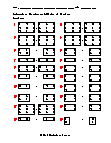Receive free math worksheets via email:

The term "calculate" originates form the Greek word kalyz, which means pebble or small stone. So, why is this the basis for our advanced math concepts? Simple: a long time ago, the Greeks used small stones to do simple calculations. Every math whiz knows -- you gotta start somewhere!= free= members onlyBasic Lesson
Guides students through solving the sum of matrices.
Standard: MATH 3View lessonIntermediate Lesson
Demonstrates how to calculate the sum of three row-column matrices.
Standard: MATH 3View lessonIndependent Practice 1
A really great activity for allowing students to understand the concept of Addition of Matrices .
Standard: MATH 3View worksheetIndependent Practice 2
Students find the Addition of Matrices in assorted problems. The answers can be found below.
Standard: MATH 3View worksheetStudents are provided with problems to achieve the concepts of Addition of Matrices.
Standard: MATH 3View homeworkThis tests the students ability to evaluate Addition of Matrices.
Standard: MATH 3View quizAnswers for the homework and quiz.View answersAnswers for lessons and both practice sheets.View answersHow to Reduce Anxiety and Nervousness on Math Tests
Sweaty palms, dry mouth, heart palpitations and to top it all up, your mind goes totally blank instantly.View Math ArticleWhy getting the correct math answer is not always important.
Clearly, showing one's work provides an insight into where problem areas may lie.View Math Article